## Induction Motor Speed Control Methods:

The only way to control the speed of a synchronous motor is to control the input frequency and voltage such as to keep V/f constant. The Induction Motor Speed Control, on the other hand, can be controlled by the following means:

1. Stator Voltage Control
2. Frequency Control
3. Slip Control

### 1. Stator Voltage Control:

This is applicable for small motors and for fan-type load, where the load torque increases with speed. As already mentioned earlier, there are two ways of controlling the rms value of the stator voltage—phase-control and integral cycle control.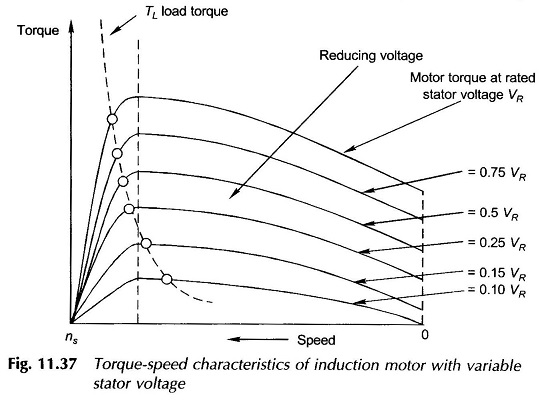The torque-speed characteristics of an induction motor with stator voltage control are drawn in Fig. 11.37. The maximum torque reduces as the square of the voltage.

The figure also illustrates that speed regulation is only possible for a load whose torque decreases with speed. Even then speed regulation is not achieved for low speeds—here the speed drops off sharply.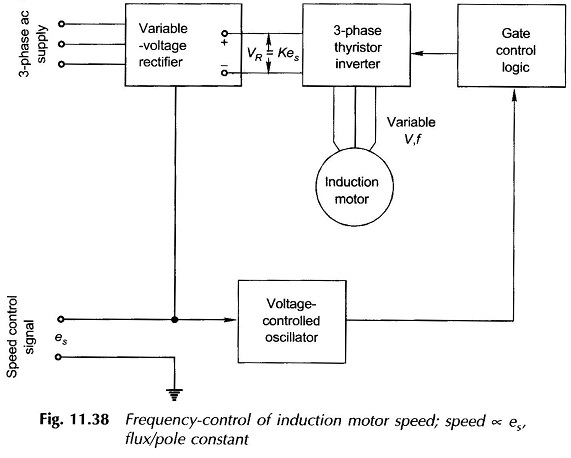### 2. Frequency Control:

Here the input frequency is varied by means of an inverter; for subnormal frequency the stator voltage is also varied to keep V/f constant which maintains the resultant flux/pole in the air-gap. A typical control system utilizing frequency control is schematically illustrated in Fig. 11.38. Because of large capital outlay, this system is justified only for drives wherein the rugged, maintenance-free characteristics of the induction motor are essential. Otherwise, a dc motor with converter control is the logical and cheap alternative. The heart of the frequency control scheme is the 3-phase thyristor inverter.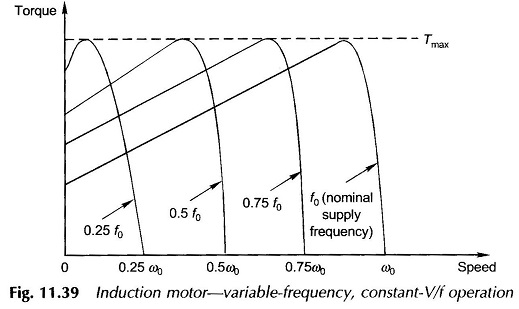The torque-speed characteristics of variable-frequency, constant-V/f control were illustrated in Fig. 11.39. It may be seen that the maximum torque does not alter because the flux/pole is kept at a fixed value.

### 3. Slip Control:

It is already known that subsynchronous control of the Induction Motor Speed is possible by introducing a variable resistance in the rotor circuit of a wound-rotor induction motor. This method provides a wide range of speed and a good starting torque as illustrated in Fig. 11.40. The maximum torque in this case remains constant. Good regulation of speed is achievable for both constant-torque and fan-type loads.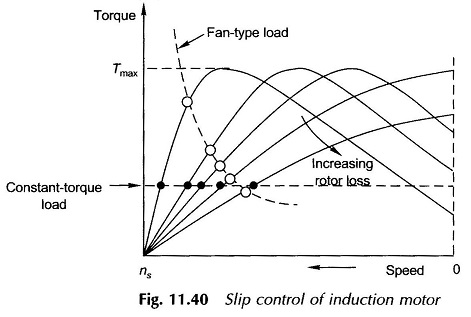The greatest draw-back of this Induction Motor Speed Control is its poor efficiency because of power wasted in the external rotor resistance.

The slip control method is based upon the general principle that the induction motor slip increases as more electric power loss occurs on the rotor circuit. Variable rotor circuit loss can be achieved by the scheme of introducing a controlled converter in the rotor circuit feeding a fixed resistance as shown in Fig. 11.41.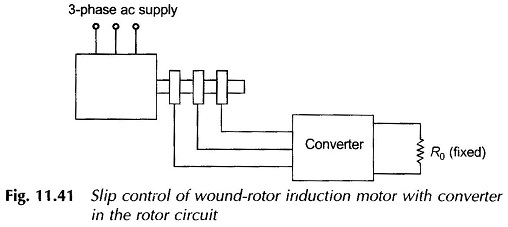Rather than wasting the slip energy in external resistance, it can be recovered and fed back to supply, thereby removing the disadvantage of low efficiency of the slip-control method.

Scroll to Top